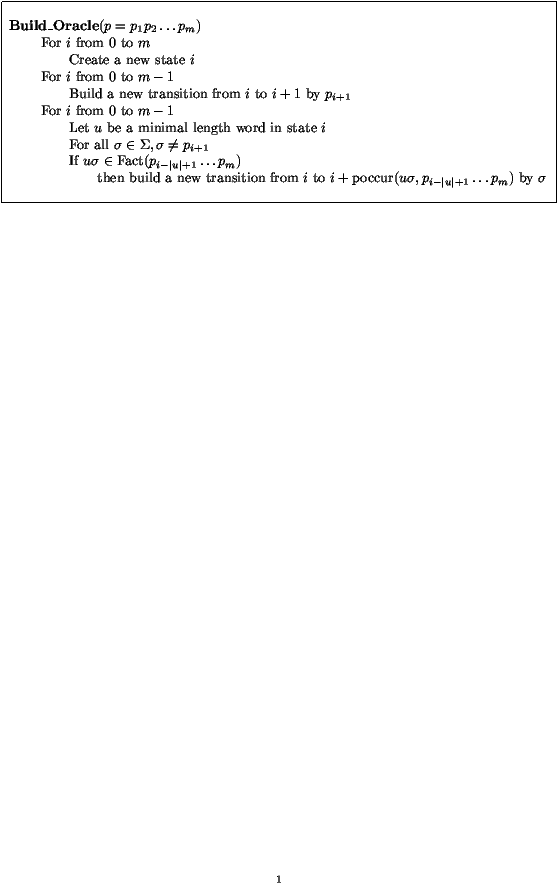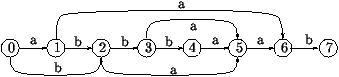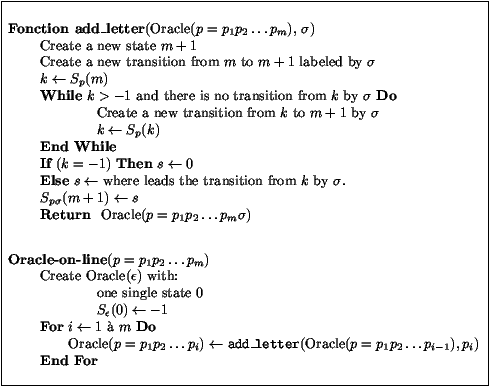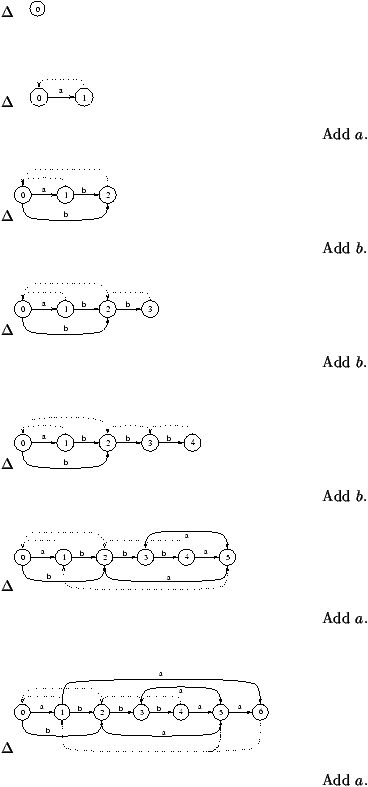Factor Oracle, Suffix Oracle

Matthieu Raffinot

Institut Gaspard-Monge, Université de Marne-la-Vallée

Algorithms Seminar

October 4, 1999

[summary by Alain Denise and Matthieu Raffinot]

A properly typeset version of this document is available in postscript and in pdf.

If some fonts do not look right on your screen, this might be fixed by configuring your browser (see the documentation here).

Abstract
The aim of this work is to design efficient algorithms for string matching. For this purpose, we introduce a new kind of automaton: the factor oracle, associated with the string p to be recognized in a text. This leads to simple algorithms which are as efficient in time as already known ones, while using less memory. This is a joint work with Cyril Allauzen and Maxime Crochemore.

## 1   Introduction

The efficiency of string matching algorithms depends on the underlying automaton which represents the string p to be found in the text. Ideally, this automaton A should satisfy the following properties:
1. A is acyclic;
2. A recognizes at least the factors of p;
3. A has the fewer states as possible;
4. A has a linear number of transitions according to m, the length of p. (Such an automaton has at least m+1 states.)
The suffix or factor automaton [3, 5] satisfies 1., 2., and 4. but not 3. whereas the subsequence automaton  satisfies 1., 2., and 3. but not 4. We present in Section 2 an intermediate structure called factor oracle: an automaton with m+1 states that satisfies all the above requirements. Section 3 is devoted to the study of a string matching algorithm based on the factor oracle.

## 2   Construction of the Factor OracleFigure 1: High-level construction of the Oracle.

The factor oracle of a word p=p1p2... pm, denoted Oracle(p), is the automaton built by the algorithm Build_Oracle (Figure 1). All the states of the automaton are final. Figure 2 gives the factor oracle of the word p=abbbaab. On this example, the reader will notice that the word aba is recognized whereas it is not a factor of p.Figure 2: Factor oracle of abbbaab.

Here are some notations which are used in the following. The set of all prefixes (resp. suffixes) of p is denoted by Pref(p) (resp. Suff(p)). The word prefp(i) is the prefix of length i of p for 0£ i£ m. For any uÎFact(p), we define
 poccur(u,p)=min { |z||z=wu and p=wuv } ,
the ending position of the first occurrence of u in p. For any uÎFact(p), we define the set
endposp(u)={ i| p=wupi+1... pm }.
Given two factors u and v of p, we write u~p v if endposp(u)=endposp(v).

The authors prove in  the following lemmas.

Lemma 1   Given a state i of Oracle(p), let uÎS* be a minimal length word among the words recognized in i. Then uÎFact(p) and i=poccur(u,p).

Corollary 1   For any state i of Oracle(p), there exists an unique minimal length word among the words recognized in state i.

We denote min(i) the minimal length word of state i.

Corollary 2   Let i and j be two states of Oracle(p) such that j<i. Then min(i) cannot be a suffix of min(j).

Lemma 2   Let i be a state of Oracle(p). Then min(i) is a suffix of any word cÎS* which is the label of a path leading from state 0 to state i.

Lemma 3   Any word wÎFact(p) is recognized by Oracle(p) in a state j£poccur(w,p).

Corollary 3   Let wÎFact(p). Every word vÎSuff(w) is recognized by Oracle(p) in a state j£poccur(w).

Lemma 4   Let i be a state of Oracle(p). Any path ending by min(i) leads to a state j³ i.

Lemma 5   Let wÎ S* be a word recognized by Oracle(p) in i. Any suffix of w is recognized in a state j£ i.

Lemma 6   The number TOr(p) of transitions in Oracle(p=p1p2... pm) satisfies m £ TOr(p) £ 2m-1.

The high-level construction of the factor oracle is equivalent to the on-line algorithm given in Figure 3. An example of this construction is shown in Figure 4.Figure 3: On-line construction of Oracle(p=p1p2... pm).

##### Exemple
The on-line construction of Oracle(abbbaab) is given Figure 4.Figure 4: On-line construction of Oracle(abbaba).

## 3   String Matching

The authors replace the suffix automaton with a factor oracle in the BDM (for backward dawg matching) [4, 6], obtaining the BOM (for backward oracle matching) algorithm.

The BOM algorithm consists in shifting a window of size m on the text. For each new position of this window, the factor oracle of the mirror image of p is used to search the suffix of the window from right to left. The basic idea is that if this backward search fails on a letter s after the reading of a word u then s u is not a factor of p and the beginning of the window can be shifted just after s. The worst-case complexity of BOM is O(nm).

The average complexity of the original BDM is in O(nlog|S|(m)/m) under a uniform Bernoulli model. In view of the experimental results (see ), the authors claim that their new BOM algorithm is also optimal on average:
Conjecture 1   Under a model of independence and equiprobability of letters, the BOM algorithm has an average complexity of O(nlog|S|(m)/m).
The authors show in  how to obtain a linear (in n) worst case algorithm from the BOM.

## References


Allauzen (Cyril), Crochemore (Maxime), and Raffinot (Mathieu). -- Oracle des facteurs, oracle des suffixes. -- Technical Report n°99-08, Institut Gaspard-Monge, Université de Marne-la-Vallée, 1999. Available from `http://www-igm.univ-mlv.fr/~raffinot/ftp/IGM99-08.ps.gz`.


Baeza-Yates (Ricardo A.). -- Searching subsequences. Theoretical Computer Science, vol. 78, n°2 (Part A), 1991, pp. 363--376.


Blumer (A.), Blumer (J.), Haussler (D.), Ehrenfeucht (A.), Chen (M. T.), and Seiferas (J.). -- The smallest automaton recognizing the subwords of a text. Theoretical Computer Science, vol. 40, n°1, 1985, pp. 31--55. -- Special issue: Eleventh international colloquium on automata, languages and programming (Antwerp, 1984).


Crochemore (M.), Lecroq (T.), Czumaj (A.), Gasieniec (L.), Jarominek (S.), Plandowski (W.), and Rytter (W.). -- Speeding up two string-matching algorithms. Algorithmica, vol. 12, n°4-5, 1994, pp. 247--267.


Crochemore (Maxime). -- Transducers and repetitions. Theoretical Computer Science, vol. 45, n°1, 1986, pp. 63--86.


Crochemore (Maxime) and Rytter (Wojciech). -- Text algorithms. -- The Clarendon Press Oxford University Press, New York, 1994, xiv+412p.

This document was translated from LATEX by HEVEA.